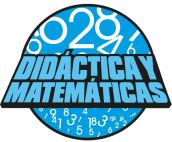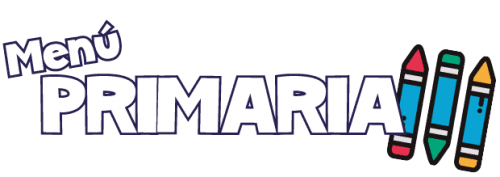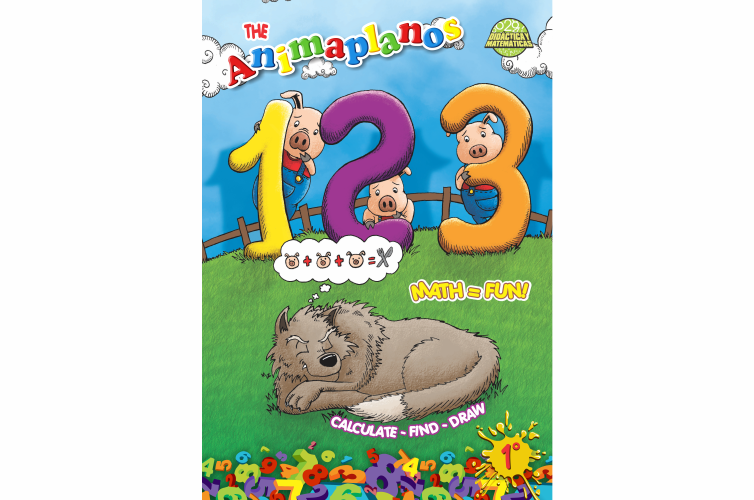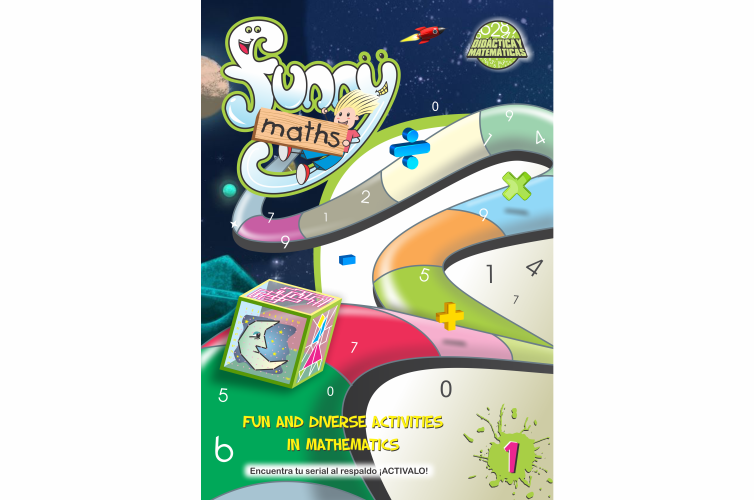Didáctica Y MatemáticasMatemáticas en InglesSTRATEGIES TO DEVELOP PERCEPTION AND NUMERICAL CALCULUS6 to 10 years of ageThe content aims to provide strategies to practice the development of mental calculus by solving numerical operations and problems. Students find motivation as they calculate, find and draw attractive images on a 100-point grid.STRATEGIES TO DEVELOP PERCEPTION AND NUMERICAL CALCULUS6 to 10 years of ageThe content aims to provide strategies to practice the development of mental calculus by solving numerical operations and problems. Students find motivation as they calculate, find and draw attractive images on a 100-point grid.